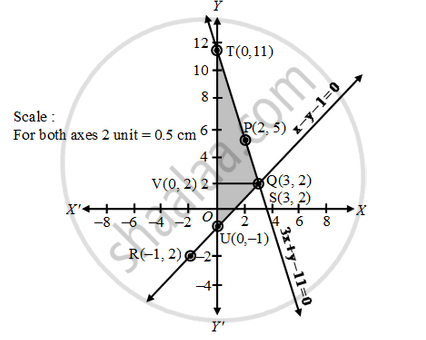# Solve the Following System of Linear Equations Graphically; 3x + Y – 11 = 0 ; X – Y – 1 = 0 Shade the Region Bounded by These Lines and Also Y-a - Mathematics

Sum

Solve the following system of linear equations graphically; 3x + y – 11 = 0; x – y – 1 = 0 Shade the region bounded by these lines and also y-axis. Then, determine the areas of the region bounded by these lines and y-axis.

#### Solution

We have,

3x + y – 11 = 0 and x – y – 1 = 0

(a)Graph of the equation 3x + y – 11 = 0

We have, 3x + y – 11 = 0

⇒ y = – 3x + 11

When, x = 2, y = –3 × 2 + 11 = 5

When, x = 3, y = – 3 × 3 + 11 = 2

Plotting the points P (2, 5) and Q(3, 2) on the graph paper and drawing a line joining between them, we get the graph of the equation 3x + y – 11 = 0 as shown in fig.

(b) Graph of the equation x – y – 1 = 0

We have,

x – y – 1 = 0

y = x – 1

When, x = – 1, y = –2

When, x = 3, y = 2

Plotting the points R(–1, –2) and S(3, 2) on the same graph paper and drawing a line joining between them, we get the graph of the equation x – y – 1 = 0 as shown in fig.You can observe that two lines intersect at Q(3, 2). So, x = 3 and y = 2. The area enclosed by the lines represented by the given equations and also the y-axis is shaded.

So, the enclosed area = Area of the shaded portion

= Area of ∆QUT = 1/2 × base × height

= 1/2 × (TU × VQ) = 1/2 × (TO + OU) × VQ

= 1/2 (11 + 1) 3 = 1/2 × 12 × 3 = 18 sq.units.

Hence, required area is 18 sq. units.

Concept: Graphical Method of Solution of a Pair of Linear Equations
Is there an error in this question or solution?

#### APPEARS IN

RD Sharma Class 10 Maths
Chapter 3 Pair of Linear Equations in Two Variables
Exercise 3.2 | Q 28 | Page 30